Designing of Turing Machines

# Designing of Turing Machines - Theory of Computation - Computer Science Engineering (CSE)

Part II. Designing of Turing Machines

3 Designing of TM

Following are the basic guidelines for designing a TM.
1. The fundamental objective of scanning a symbol in the tape is to know what to do in the future. TM must remember the past symbols scanned. TM can remember this by going to the next unique state.
2. The number of states must be minimised. This is achieved by changing the states only when there is a change in the written symbol or when there is a change in the movement of the head.

Following examples show designing of TMs.

Example 1:
Design a turing machine over {a} to accept the language L={an|n is odd}.
The initial instant of the TM is shown below. The input tape contains aaa followed by blanks. The TM is initially in state q0 and head points to the first a of the input string w.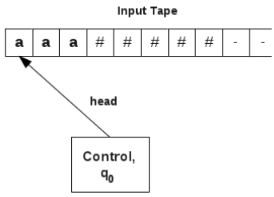The working of the TM is as follows:
In state q0, head reads symbol a from the current cell and
1. keeps the contents of the current cell as a,
2. changes state of the TM to q1, and
3. moves to the next cell on the right side.
The corresponding transition function is,
δ(q0, a) = (q1, a, R)

In state q1, head reads symbol a from the current cell and
1. keeps the contents of the current cell as a,
2. changes the state of the TM to q0, and
3. moves to the next cell on the right side.

The corresponding transition function is,
δ(q1, a) = (q0, a, R)

Thsi process continues till a blank symbol, # is reached.
If the blank symbol is reached in state q1, it indicates that input string contains an odd number of a’s.
If the blank symbol is reached in state q0, it indicates that input string contains an even number of a’s.
Hence, qis the final state.

Transition table for the TM is,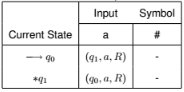Consider the processing of string, aaa using the above TM,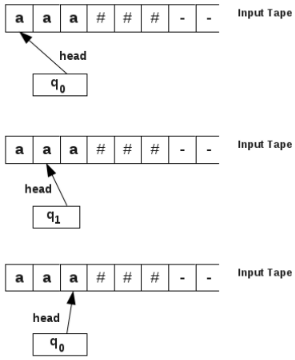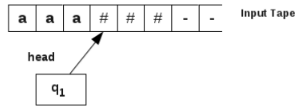The TM halts at state q1. qis a final state. So the string aaa is accepted.

Consider the processing of string, aaaa using the above TM,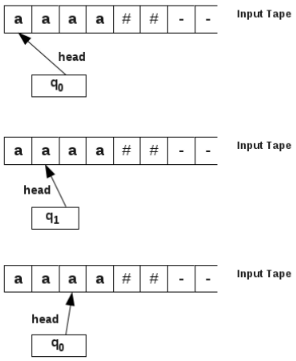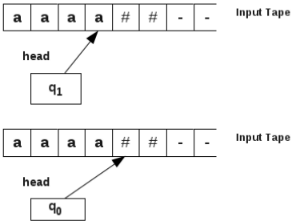The TM halts at state q0. qis not a final state. So the string aaaa is not accepted.

Example 2:
Design a turing machine over {a} to accept the language L={an|n is even}. The initial instant of the TM is shown below. The input tape contains aaaa followed by blanks. The TM is initially in state
q0 and head points to the first a of the input string w.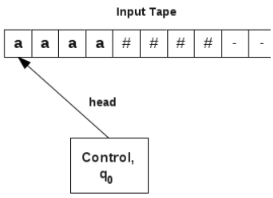The working of the TM is as follows:
In state q0, head reads symbol a from the current cell and
1. keeps the contents of the current cell as a,
2. changes state of the TM to q1, and
3. moves to the next cell on the right side.
The corresponding transition function is,
δ(q0, a) = (q1, a, R)

In state q1, head reads symbol a from the current cell and
1. keeps the contents of the current cell as a,
2. changes the state of the TM to q0, and
3. moves to the next cell on the right side.
The corresponding transition function is,
δ(q1, a) = (q0, a, R)

Thsi process continues till a blank symbol, # is reached.
If the blank symbol is reached in state q0, it indicates that input string contains an even number of a’s.
If the blank symbol is reached in state q1, it indicates that input string contains an odd number of a’s.
Hence, qis the final state.

Transition table for the TM is,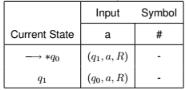Consider the processing of string, aaaa using the above TM,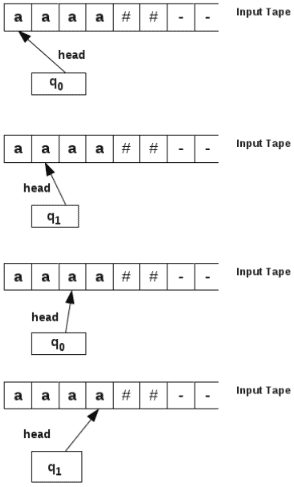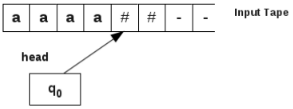The TM halts at state q0. qis a final state. So the string aaaa is accepted.

Example 3:
Design a Turing machine over {a, b} to accept the language L={anbn|n ≥ 1 }.
Example strings in this language are ab, aabb, aaabbb, aaaabbbb,.........
Let the string be aaaabbbb.
String recognition process of the Turing machine is as follows:
Initially, TM is in state q0 and head points to first a in the string.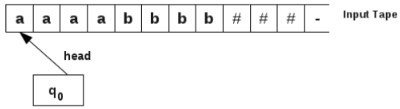1. Read the first symbol a of the string and convert this a to blank (#).
2. The head keeps moving towards right till the input string is crossed and the first blank symbol after the string is reached.
3. From this #, turn one cell left and reach the last symbol of the input string. If it is 1, convert it to #. After performing the first three steps, input tape with aaaabbbb###, will contain #aaabbb####. That is, leftmost a is cancelled with rightmost b.
4. Now after converting the last b to #, the head keeps moving towards left till a # is reached, turn towards right and converts the first available a to #, and keeps moving towards right till a # is reached. It then turns left and converts the first available b to # and keeps moving towards left. After step 4, input tape will contain ##aabb#####. This marks the cancellation of the next leftmost a by the next rightmost
b.
5. The head keeps repeating step 4 till all the a’s are cancelled by teh corresponding b’s.
6. If the cancellation process is successfully completed, then the string is accepted.
7. If there are remaining uncancelled a’s or b’s, then the input string is not accepted.

Transition table corresponding to the TM is given below: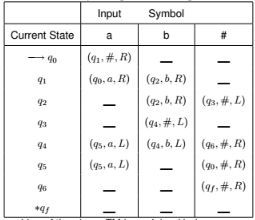The working of the above TM is explained below:
1. The head reads the first symbol of the input string in state q0 and does the following:
a. If the symbol is # or b, then TM halts. If the first symbol is #, then it is a null string and does not belong to the language. If the symbol is ’b’, then it is also an invalid string. Thus, q0 is a non-final state.
b. If the first symbol is ’a’, then head converts this input symbol to #, changes state to q1, and moves towards right. δ(q0, a) = (q1, #, R)

2. In state q1, head does the following:
a. If the head is pointing to input symbol a, then symbol ’a’ remains unchanged, state q1 remains unchanged, and head moves to next cell on the right side. δ(q1, a) = (q1, a, R)
b. If the head is pointing to input symbol b, then symbol ’b’ remains unchanged, state q1 changes to state q2, and head moves to next cell on the right side. δ(q1, b) = (q2, b, R)
In state q1, head simply passes over all the 0s of the input string from left to right and changes to state qas soon as symbol b is received.

3. In state q2, head does the following:
a. If the head is pointing to input symbol b, then symbol ’b’ remains unchanged, state qremains unchanged, and head moves to next cell on the right side. δ(q2, b) = (q2, b, R)
b. If the head is pointing to symbol #, then symbol ’#’ remains unchanged, state q2 changes to state q3, and head moves to cell on the left side. δ(q2, #) = (q3, #, L)
In state q2, head simply passes over all the b’s of the input string from left to right and changes to state q3 as soon as symbol # is received and turns towards left.

4. In state q3, head does the following:
a. If the head is pointing to input symbol b, then symbol ’b’ is replaced with #, state q3 changes to q4, and head moves to next cell on the left side. δ(q3, b) = (q4, #, L)
In state q3, symbol ’b’ is replaced with # in response to the ’a’ symbol replaced with # in state q0.

5. In state q4, head does the following:
a. If the head is pointing to input symbol b, then symbol ’b’ remains unchanged, state q4 remains unchanged, and head moves to next cell on the left side. δ(q4, b) = (q4, b, L)
b. If the head is pointing to symbol a, then symbol ’a’ remains unchanged, state q4 changes to state q5, and head moves to cell on the left side. δ(q4, a) = (q5, a, L)
c. If the head is pointing to symbol #, then symbol ’#’ remains unchanged, state q4 changes to state q6, and head moves to cell on the right side. δ(q4, #) = (q6, #, R). This indicates that all a’s have been cancelled.
In state q4, head simply passes over all the b’s of the input string from right to left and changes to state q5 as soon as symbol a is received.

6. In state q5, head does the following:
a. If the head is pointing to input symbol a, then symbol ’a’ remains unchanged, state q5 remains unchanged, and head moves to next cell on the left side. δ(q5, a) = (q5, a, L)
b. If the head is pointing to symbol #, then symbol ’#’ remains unchanged, state q5 changes to state q0, and head moves to cell on the right side. δ(q5, #) = (q0, #, R)
In state q5, head simply passes over all the a’s of the input string from right to left and changes to state q0 as soon as symbol # is received.

7. In steps 1-6, leftmost a and rightmost b cancel out each other. This is done by converting them to blanks. This is repeated till all the a’s are cancelled by the corresponding b’s.
When all a’s are cancelled by the respective b’s, head will point ot a # symbol in state q6 and moves to final state where it halts and it indicates that the string is accepted.

8. In state q6, head does the following:
a. If the head is pointing to input symbol #, then it indicates that all the a’s have been successfully matched with the corresponding b’s. Hence the TM changes state to qf and halts.It indicates taht the string is accepted. δ(q6, #) = (qf , #, R)
b. If the head is pointing to symbol b, this shows that the number of b’s is more than the number of a’s and hence the TM halts in state q6 to indicate the non-acceptance of the string.
Let the input string is aaabbb.
TM processes the string as follows: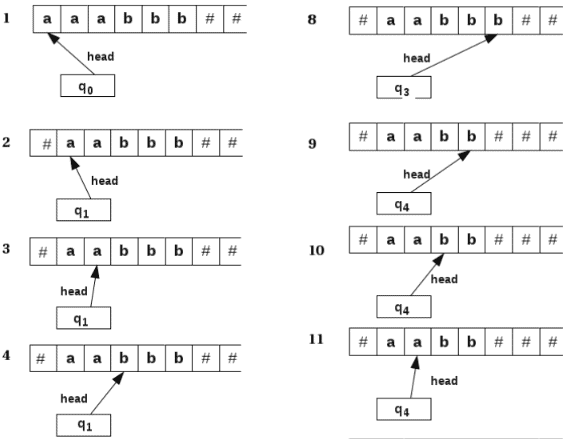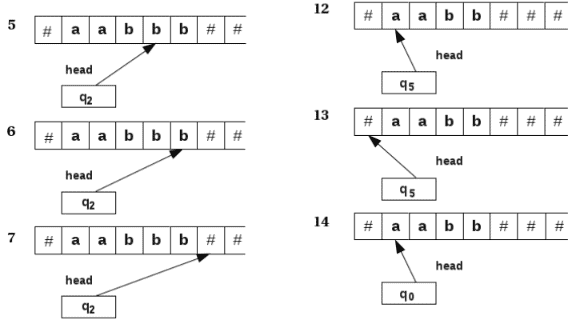After the 14th step leftmost a is cancelled with the rightmost b. This process is repeated and finally we get all #s and TM will reach state qf . Then the string aaabbb is accepted by the above TM.

Example 4:
Design a Turing machine over {a, b} to accept the language L={wwR|w ∈ (a, b)+ }.
Example strings in this language are aa, abba, bb, baab, ababbbbaba, ........

String recognition process of the Turing machine is as follows:
1. Initially, TM is in state q0 and head points to first symbol in the string.
2. The symbol may be a or b. Aftyer reading this symbol, this symbol is replaced with a # and keeps moving towards right till the whole of the string is crossed and the first # after  the string is reached.
3. From this blank, the head turns one cell left and reaches the last symbol of the input string. If this symbol matches with the first symbol of the input string, it converts it into a #.
4. Steps 1-3 match the first and last symbols of the input string. If this matching is successful, the head traverses back and crosses the string to reach the first #. From this #, it turns towards right and matches the leftmost and rightmost symbols of the remaining string. If the matching is successful, then the symbols are converted to #s.
5. The process repeats till the symbols are matched.
6. To keep track of the symbol to be matched, if symbol ’a’ is received in state q0, then head enters state q1, and if symbol ’b’ is received, then head enters state q2.

Transition table corresponding to the Turing machien is given below: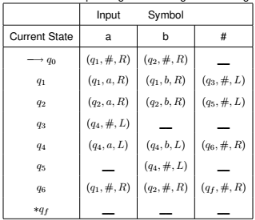Let the input string is abaaba.
TM processes the string as follows: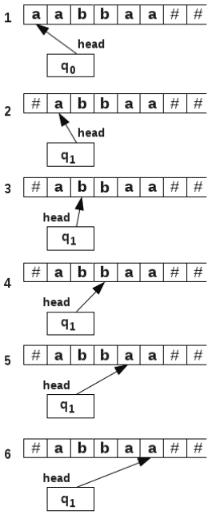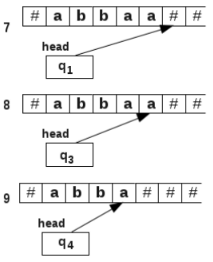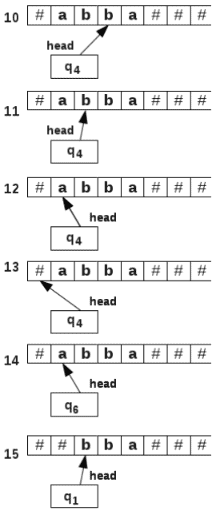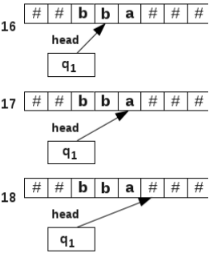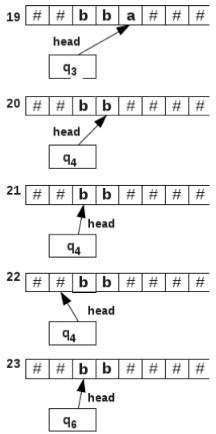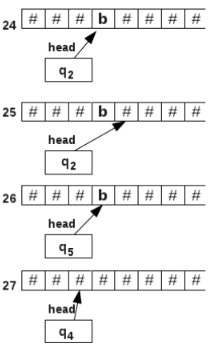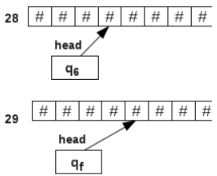Example 5:
Design a Turing machine over {a, b, c} to accept the language L={anbncn|n ≥ 1 }.
Example strings in this language are abc, aabbcc, aaabbbccc, aaaabbbbcccc, ....

String recognition process of the Turing machine is as follows:
1. Initially, TM is in state q0 and head points to first symbol, a in the string. a is replaced with a #, changes state to q1, and moves towards right.
2. In state q1, the head continues to move towards right till all the a’s are passed over and the first ’b’ is obtained. Now ’b’ is replaced with ’x’, changes state to q2, and again moves towards right.
3. In state q2, the head continues to move towards right till all the b’s are passed over and the first ’c’ is obtained. Now ’c’ is replaced with ’y’, changes state to q3, and turns back towards left.
4. In steps 1-3, each symbol ’a’ is matched with the corresponding symbols ’b’ and ’c’. In state q3, head continues to move towards left and passes over the whole string to reach the blank symbol, #. At #, it turns right and changes state to q4.
5. In state q4, head reads symbol ’a’, replaces it with a #, changes to state q1and moves towards right.
6. Now steps 1-3 are repeated to match the symbols a, b and c. this process continues till the symbol ’x’ is obtained in state q4. This shows that all a’s were replaced with #’s. Now if the string is valid, no ’b’ should be available in state q4.
7. In state q4,head continues to move towards right till all the x’s are passed over and no symbol, ’b’ is obtained. If ’b’ is found, it indicates a mismatch in the number of ’a’ and ’b’ symbols. TM halts in state q4 to indicate the rejection of the string.
If no ’b’ is encountered, and if symbol ’y’ is obtained, then it changes state to q5, and moves towards right.
8. In state q5,head continues to move towards right till all the y’s are passed over and no symbol, ’c’ is obtained. If ’c’ is found, it indicates a mismatch in the number of ’a’ and ’c’ symbols. TM halts in state q5 to indicate the rejection of the string.
If no ’c’ is encountered, and if symbol ’#’ is obtained, then it changes state to qf , and moves towards left.
9. In state qf , TM halts to indicate the acceptance of the string.
Transition table for the TM is shown below: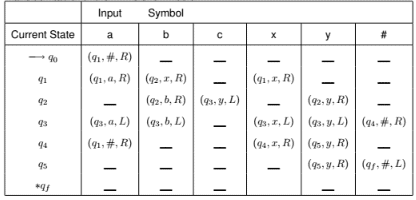Exercises:
1. Design a TM that accepts the language L = {0n|n is a multiple of 3}.
2. Design a TM that accepts the language L = {anb2n|n > 0}.
3. Design a TM over {a,b,c} to accept the language L = {wcw|w ∈ (a|b)*}.
4. Design a TM over {a,b} to accept the language L = {ww|w ∈ (a|b)*}

The document Designing of Turing Machines | Theory of Computation - Computer Science Engineering (CSE) is a part of the Computer Science Engineering (CSE) Course Theory of Computation.
All you need of Computer Science Engineering (CSE) at this link: Computer Science Engineering (CSE)

## Theory of Computation

18 videos|56 docs|44 tests

## FAQs on Designing of Turing Machines - Theory of Computation - Computer Science Engineering (CSE)

 1. What is a Turing Machine?Ans. A Turing Machine is a theoretical computing device that can simulate any algorithmic computation. It consists of an input tape, a tape head that can read and write symbols on the tape, a control unit to interpret instructions, and a set of rules to determine the machine's behavior. Turing Machines are used in computer science to study the limits of computation and the foundations of algorithms.
 2. What is the significance of Turing Machines in computer science engineering?Ans. Turing Machines play a crucial role in computer science engineering as they are used to study the fundamentals of computation. They help in understanding the limits of what can be computed and provide insights into the complexity of algorithms. By analyzing Turing Machines, engineers can design efficient algorithms, solve complex computational problems, and develop reliable software systems.
 3. How does a Turing Machine work?Ans. A Turing Machine works by reading symbols from an input tape, modifying the tape according to a set of rules, and transitioning between different states. The machine starts at an initial state and moves its tape head based on the current symbol and state. It then performs specific actions, such as writing symbols, moving the tape head, or changing its state, as defined by the rules. This process continues until the machine reaches a halting state or an infinite loop.
 4. Can a Turing Machine solve any computational problem?Ans. Yes, a Turing Machine can theoretically solve any computational problem that can be expressed as an algorithm. It can simulate the behavior of any computer program, given enough time and resources. However, it's important to note that while a Turing Machine can solve any problem in theory, practical limitations such as time and memory constraints may make certain problems infeasible to solve in practice.
 5. What are the limitations of Turing Machines?Ans. Turing Machines have certain limitations. They are unable to solve problems that are undecidable or unsolvable, such as the halting problem. Additionally, Turing Machines do not capture the parallelism and concurrency present in modern computing systems. They also do not consider the physical constraints and limitations of real-world computers, making them an idealized model rather than a practical implementation. Various extensions to Turing Machines, such as quantum Turing Machines, have been proposed to address some of these limitations.

## Theory of Computation

18 videos|56 docs|44 testsExplore Courses for Computer Science Engineering (CSE) examSignup to see your scores go up within 7 days! Learn & Practice with 1000+ FREE Notes, Videos & Tests.
10M+ students study on EduRev
Track your progress, build streaks, highlight & save important lessons and more!
Related Searches

,

,

,

,

,

,

,

,

,

,

,

,

,

,

,

,

,

,

,

,

,

;